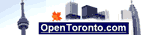Entrar °F o °C para la conversion :

Seleccionar un tipo de la conversion :

Opciones:

 Tabla de conversion de F a C: -30 F = -34.444 C -20 F = -28.889 C -10 F = -23.333 C   0 F = -17.778 C 10 F = -12.222 C 20 F = -6.667 C 30 F = -1.111 C 40 F = 4.444 C 50 F = 10 C 60 F =15.556 C 70 F = 21.111 C 80 F = 26.667 C 90 F = 32.222 C 100 F = 37.778 C Tabla de conversion de C a F: -30 C = -22 F -20 C = -4 F -10 C = 14 F   0 C = 32 F 10 C = 50 F 20 C = 68 F 30 C = 86 F 40 C = 104 F 50 C = 122 F 60 C = 140 F 70 C = 158 F 80 C = 176 F 90 C = 194 F 100 C = 212 F

Deutsch   English   Espanol   Francais   Italiano   Portuguese

Calculators:
MPG calculator
L/100km fuel calculator
L/100km to MPG calculator
MPG to L/100km conversion
Miles to km conversion
mph to kmh conversion
Gallon to Litre Conversion
Pounds to kilograms conversion
Celsius to Fahrenheit
Fahrenheit to Celsius conversion
Recommended Daily Calorie Calculator
BMI Calculator
Ounces to grams conversion
Yards to mm conversion
Yards to meters Conversion
Feet to meters Conversion
Inches to mm Conversion
Time in Hamilton, Ontario